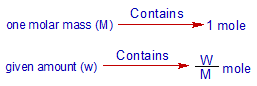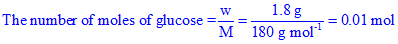# Number of moles of glucose molecules

## How many moles of glucose molecules are present in 1.8 grams of a sample of glucose?

Logic:

The molar mass, sometimes referred to as molecular weight, is the mass of one mole of a substance and is usually expressed in grams.

Therefore,Therefore we can write,What is the molar mass of glucose (C6H12O6)? How to calculate....?

The molar mass of glucose can be calculated from the molar masses of individual atoms present in it.

From the molecular formula, C6H12O6, one can find there are 6 carbon atoms, 12 hydrogen atoms and 6 oxygen atoms in one molecule of glucose.

We know that:

Molar mass of C = 12 g mol-1

Molar mass of H = 1 g mol-1

Molar mass of O = 12 g mol-1

Therefore,

Molar mass of C6H12O6=(6x12) + (12x1) + (6x16) = 72 + 12 + 96 = 180 g mol-1

Plugin the values to get the number of moles of glucose as follows:How to calculate the number of molecules?

It is also possible to get the number of molecules in the given sample by multiplying the number of moles with Avogadro number, NA

Thus,

the number of molecules = n.NA

Where NA = 6.022 x 1023

In above glucose sample,

the no. of glucose molecules = 0.01 x 6.022 x 1023 = 6.022 x 1021

Remember that one mole of any substance contains Avogadro number of particles (may be atoms or molecules or ions etc.). If the substance is molecular, the number molecules will be equal to 6.022 x 1023. If we take atomic substance, then the number of atoms in one mole is equal to Avogadro number.

### Related questions

1) How many molecules of of sucrose (C12H22O11) are present in 34.2 grams of pure sugar?

Hint: Get the number of moles and multiply the outcome with avagodro number.

2) Calculate the number of atoms present in 54 grams of aluminium.

3) How many atoms are present in 18 grams of water?

4) How many NaCl units are present in 1 gram of common salt? Consider the sample is 100% pure.

5) Calculate the number of moles of CaCO3 present in 10 grams of 80% pure sample of limestone.

Hint: Find the number of moles and multiply with 80/100.

6) How many electrons are present in 9.1 x 10-29 Kg?

Hint: What is the mass of one electron?

7) What is the charge on one mole of electrons?

Hint: What is the charge on one electron?

8) What is the weight of 3 moles of O2 gas?

9) Calculate the molar mass of SO2.